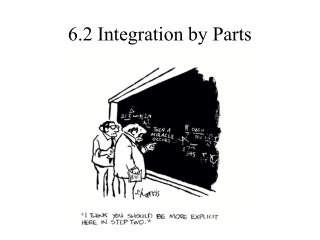DownloadDownload Presentation6.2 Integration by Parts

# 6.2 Integration by Parts

Download Presentation## 6.2 Integration by Parts

- - - - - - - - - - - - - - - - - - - - - - - - - - - E N D - - - - - - - - - - - - - - - - - - - - - - - - - - -
##### Presentation Transcript

1. 6.2 Integration by Parts

2. First, start with the Product Rule for differentiation. Integrate both sides of the equation to obtain: Simplify, The Fundamental Theorem of Calculus tells us that if we take the derivative of the integral of a function, then we are left with the original function. The derivative and the integral “cancel” each other out. and by manipulating the equation we get…

3. This is the formula for Integration by Parts. Just as the Substitution Method could be thought of as the Chain Rule for integration, Integration by Parts could also be thought of as the Product Rule for integration. When using this formula, we need to choose which part of the integrand (what we are taking the integral of) is u, and which part of it is dv. When choosing u and dv, we want to find a u that will be simplified after we take its derivative, and a dv that won’t be too complex after integrating it.

4. Example 1 First, pick u and dv. Now take the derivative of u and the integral of dv. The second integral is much easier and more familiar than the original integral. Use the formula for Integration by Parts.

5. When deciding what to choose for u, remember L I P E T. L - logarithmic function I - inverse trig function P - polynomial function E - exponential function T - trigonometry function This is usually the preference order in which you would want to choose u.

6. Example 2 Remember L I P E T. Easy right? Now what if we have a u and a dv that NEVER runs out of derivatives or integrals?…

7. Example 3  Remember we USUALLY follow LIPET, not necessarily ALWAYS. Using the formula: Simplify to get: Integrate by parts…again? Integrate by parts again.

8.   But wait, haven’t we seen this somewhere before? What were we originally taking the integral of again? Oh yeah! Add the integral to both sides to get: Divide both sides by 2 and…

9. This is called solving for the unknown integral. When we have a product in which both factors never run out of integrals or derivatives, we can solve for the unknown integral by using the Integration by Parts formula repeatedly until the original integral shows up in the problem again. When solving for the unknown integral, it is usually a good idea to stay consistent with your u and dv. That is, if you chose u to be sin x in your first integral, you would want to choose the trig function (in this case cos x) to be u for your following integrals.

10. Example 4 Notice that when we choose u and dv the second time, u the second time is du from the first, and dv this time is v from the first time.

11. If we were to use Integration by Parts on the last integral we would have: u = 2 dv = -cos x dx du = 0 v = -sin x Again u this time is du from the previous integral, and dv this time is v from the previous integral. First of all, finishing this integral we have: Keep this answer handy and let’s take a different look at this problem.

12. But this time, let’s take the derivative of u all the way through and integrate dv for everytime we differentiate u. We’ll choose the same u and dv as we did the first time. Notice that when we keep taking the derivative of u it eventually goes to 0, and dv never runs out of integrals. u dv (+) x2 cos x If we multiply each u by its respective v and we switch the signs each time we add each part together we get… ( - ) 2x sin x (+) 2 -cos x 0 -sin x x2 sin x + 2x cos x - 2 sin x + C This method is called tabular integration. This works when the derivatives of u eventually reach 0 and the integrals of dv never end. This is much quicker than using the formula over and over again.